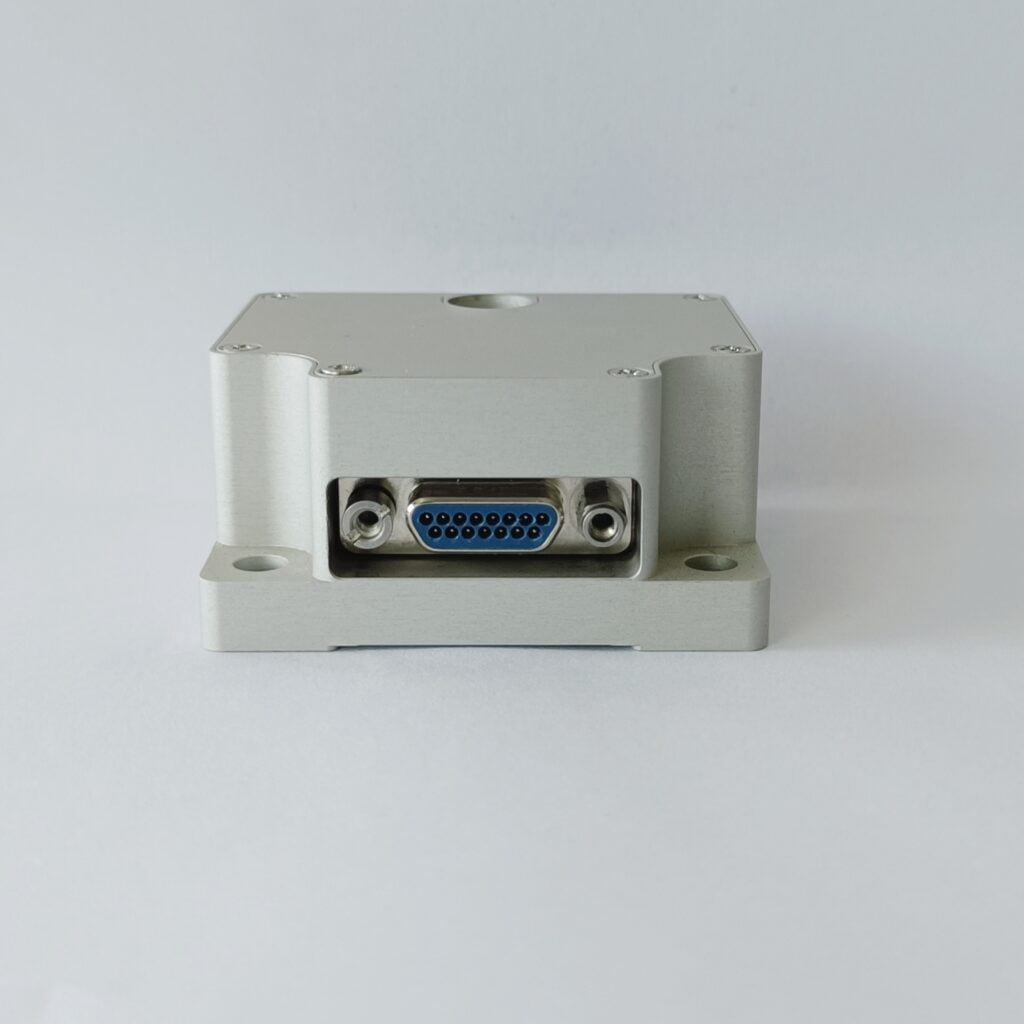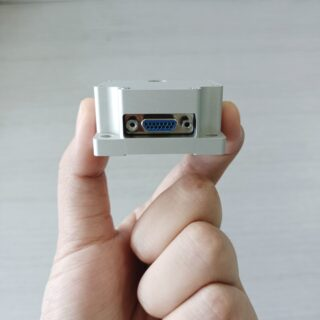Application

# Do You Know What IMU is?Gyroscope and accelerometer are the main components of IMU, and their accuracy directly affects the accuracy of inertial system. In practical work, due to various inevitable interference factors, gyroscopes and accelerometers produce errors. From the initial alignment, its navigation error increases with time, especially the position error, which is the main disadvantage of inertial navigation system. Therefore, it is necessary to use external information to realize integrated navigation, so as to effectively reduce the problem of error accumulation with time. In order to improve reliability, more sensors can be equipped for each shaft. Generally speaking, IMU should be installed on the center of gravity of the measured object.

Generally, an IMU includes three single axis accelerometers and three single axis gyroscopes. The accelerometers detect the acceleration signals of the independent three axes of the object in the carrier coordinate system, while the gyroscopes detect the angular velocity signals of the carrier relative to the navigation coordinate system, measure the angular velocity and acceleration of the object in three-dimensional space, and calculate the attitude of the object based on this. It has very important application value in navigation.

IMU is mostly used in devices that need motion control, such as cars and robots. It is also used in occasions that require precise displacement estimation with attitude, such as inertial navigation equipment of submarines, aircraft, missiles and spacecraft.Summary

Using the three-axis geomagnetic decoupling and three-axis accelerometer, it is greatly affected by the acceleration of external forces. In the environment of motion / vibration, the output direction angle error is large. This field magnetic sensor has shortcomings. Its absolute reference is the magnetic line of force of the geomagnetic field. Geomagnetism is characterized by a wide range of use, but the intensity is low, about a few tenths of a Gauss, which is very easy to be disturbed by other magnets. If the instantaneous angle of the z-axis gyroscope is combined, It can make the system data more stable. The acceleration is measured in the direction of gravity. In the absence of external force acceleration, it can accurately output the roll/pitch two axis attitude angle, and this angle will not have cumulative error, which is accurate in a longer time scale. However, the disadvantage of the acceleration sensor in measuring the angle is that the acceleration sensor actually uses MEMS technology to detect the small deformation caused by the inertial force, and the inertial force is the same as gravity in essence, so the accelerometer will not distinguish the acceleration of gravity and the acceleration of external force. When the system changes speed in three-dimensional space, its output is incorrect.

The output angular velocity of the gyroscope is an instantaneous quantity, which cannot be directly used in attitude balance. The angular velocity and time integration are required to calculate the angle. The angle change obtained is added to the initial angle to obtain the target angle. The smaller the integration time DT is, the more accurate the output angle is. However, the principle of gyroscope determines that its measurement benchmark is itself, and there is no absolute reference outside the system. In addition, DT cannot be infinitely small, so the cumulative error of integration will increase rapidly with the passage of time, resulting in the output angle inconsistent with the actual, so the gyroscope can only work in a relatively short time scale.

Therefore, on the basis of no other reference, in order to obtain a more real attitude angle, we should use the weighting algorithm to develop strengths and circumvent weaknesses, combine the advantages of the two, abandon their respective shortcomings, and design an algorithm to increase the weight of the gyroscope in a short time scale, and increase the speed weight in a longer time scale, so that the system output angle is close to the real value.

Working principle of IMU

IMU is a strapdown inertial navigation system. The system consists of three acceleration sensors and three angular velocity sensors (gyroscopes). The accelerometer is used to sense the acceleration component of the aircraft relative to the vertical line of the ground, and the velocity sensor is used to sense the angle information of the aircraft. This sub component is mainly composed of two a/d converters ad7716bs and 64K e/eprom memory X25650. The a/d converter uses the analog variables of each sensor of IMU, After being converted into digital information and calculated by CPU, the aircraft pitch angle, tilt angle and sideslip angle are finally output. The e/eprom memory mainly stores the linear curve diagram of each IMU sensor and the part number and serial number of each IMU sensor. When the part is just started, the image processing unit reads the linear curve parameters in e/eprom to provide initial information for subsequent angle calculation. Ericco provides FOG IMU and MEMS IMU solution, if you are interested, please feel free to contact us.